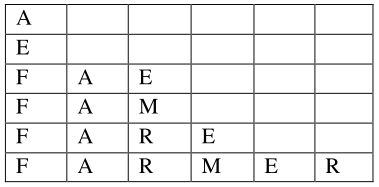# All the arrangements`
Question:

All the arrangements, with or without meaning, of the word FARMER are written excluding any word that has two $R$ appearing together. The arrangements are listed serially in the alphabetic order as in the English dictionary. Then the serial number of the word FARMER in this list is

Solution:

FARMER (6)

$\mathrm{A}, \mathrm{E}, \mathrm{F}, \mathrm{M}, \mathrm{R}, \mathrm{R}$$\frac{\lfloor}{\lfloor 2}-4=60-24=36$

$\frac{\underline{3}}{L 2}-\lfloor 2=3-2=1$

$=1$

$=2$

$=1$

______________________

77